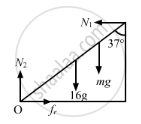Advertisement Remove all ads

# Suppose the Friction Coefficient Between the Ground and the Ladder is 0⋅540. Find the Maximum Weight of a Mechanic Who Could Go up and Do the Work Form the Same Position of the Ladder. - Physics

Sum

Suppose the friction coefficient between the ground and
the ladder of the previous problem is 0.540. Find the
maximum weight of a mechanic who could go up and do
the work from the same position of the ladder.

Advertisement Remove all ads

#### Solution

Let the maximum mass of a mechanic who could go up be m.

The system is in translation and rotational equilibrium; therefore, we have

$N_2 = 16 g + mg ...........(1)$

$N_1 = \mu N_2.........(2)$

$R_1 \times 10\cos37^\circ = 16g \times 5\sin37^\circ - mg \times 8 \sin37^\circ .........(3)$

$\Rightarrow 8 N_1 = 48g + \frac{24}{5}mg$

From eq. (2), we have

$N_2 = \frac{48 g + \frac{24}{5} mg}{8 \times 0 . 54}$Putting the value of N2 in eq.(1), we have

$16g + mg = \frac{24 . 0g + 24mg}{5 \times 8 \times 0 . 54}$

$\Rightarrow 16 + m = \frac{24 . 0 + 24m}{40 \times 0 . 54}$

$\Rightarrow m = 44 kg$

Therefore, weight of the mechanic, who can go up and do the work, should be less than 44 kg.

Is there an error in this question or solution?
Advertisement Remove all ads

#### APPEARS IN

HC Verma Class 11, 12 Concepts of Physics 1
Chapter 10 Rotational Mechanics
Q 42 | Page 198
Advertisement Remove all ads

#### Video TutorialsVIEW ALL 

Advertisement Remove all ads
Share
Notifications

View all notifications

Forgot password?